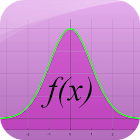Function Graph Plotter

All Android applications categories

All Android games categories# Function Graph Plotter

by: 2.2K 6.8

6.8 Users
rating

## Screenshots

Description

This free app is a function plotter and math calculator. Yor are able to draw multiple function graphs, calculate function values and value tables. You can easy calculate extremal values (minimum and maximum). It is also possible to make a numerical integration.

The following mathematical functions are available:
polynomials, rational functions, trigonometric functions, inverse trigonometric functions, hyperbolic functions, natural logarithm, exponential function and all the possible combinations of them.

Best math tool for school and college! If you are a student, it will help you to learn calculus (analysis) and algebra!

Note: Mathematical analysis is a branch of mathematics that includes the theories of differentiation, integration, measure, limits, infinite series and analytic functions. It can be applied to physics, economics and engineering.

Tags: plotter funzioni , parabel plotter , free function plotter , function plotter , plotter , function plotter profesional apk , draw fuction apk , plotter function , ploter apk

## Users review

from 2.172 reviews

"Good"

6.8